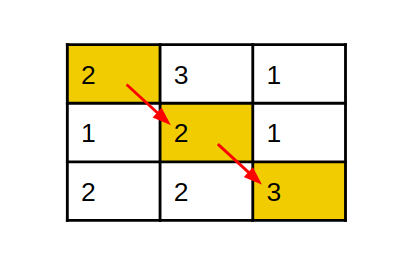9

# Minimum Cost Path

Difficulty: MEDIUM
Avg. time to solve
25 min
Success Rate
70%

Problem Statement
Suggest Edit

#### From any cell in a row, we can move to the right, down or the down right diagonal cell. So from a particular cell (row, col), we can move to the following three cells:

``````Down: (row+1,col)
Right: (row, col+1)
Down right diagonal: (row+1, col+1)
``````
##### Input Format:
``````The first line contains a single integer ‘T’ representing the number of test cases.

The first line of each test case will contain two integers ‘N’ and ‘M’ denoting the number of rows and columns, respectively.

Next ‘N’ lines contain ‘M’ space-separated integers each denoting the elements in the matrix.
``````
##### Output Format:
``````For each test case, print an integer which represents the minimum sum that can be obtained by travelling a path as described above.

Output for every test case will be printed in a separate line.
``````
##### Constraints:
``````1 <= T <= 50
1 <= N, M <= 100
-10000 <= cost[i][j] <= 10000

Where ‘T’ is the number of test cases.
Where 'N' is the number of rows in the given matrix, and 'M' is the number of columns in the given matrix.
And, cost[i][j] denotes the value at (i,j) cell in the matrix.

Time limit: 1 sec
``````
##### Sample Input 1:
``````2
3 3
2 3 1
1 2 1
2 2 3
2 3
2 3 3
5 2 1
``````
##### Sample Output 1:
``````7
5
``````
##### Explanation of sample input 1:``````In the first test case, the minimum cost path will be (0, 0) -> (1, 1) -> (2, 2), So the path sum will be (2 + 2 + 3) = 7, which is the minimum of all possible paths.

In the second test case, the minimum cost path will be (0, 0) -> (1, 1) -> (1, 2), So the path sum will be (2 + 2 + 1) = 5, which is the minimum of any possible path.
``````
##### Sample Input 2:
``````2
1 3
2 1 3
2 2
1 2
-5 3
``````
##### Sample Output 2:
``````6
-1
``````
##### Explanation for sample input 2:
``````In the first test case, there is only one possible path which is (0, 0) -> (0, 1) -> (0, 1). The path sum is (2 + 1 + 3) = 6

In the second test case, the minimum cost path will be (0, 0) -> (1, 0) -> (1, 1), So the path sum will be (1 - 5 + 3) = -1, which is the minimum of any possible path.
``````Want to solve this problem? Login now to get access to solve the problems